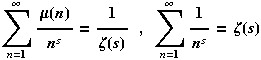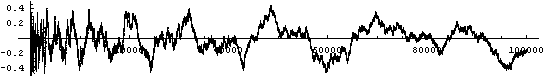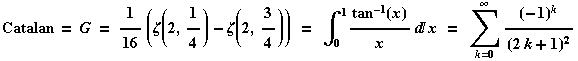Math Games The Möbius Function Ed Pegg Jr., November 3, 2003

In 1831, August Ferdinand Möbius put numbers into 3 bins, as a new type of function. His procedure is represented by the Greek letter mu (µ), and is called the Möbius function in his honor.

In the "zero" bin, Möbius placed multiples of square numbers (other than 1). {4 8 9 12 16 18 20 24 25 27 28 32 36 40 44 45 48 49 50 52 54 56 60 63 64 68} -- A013929. So, µ(48) = 0, µ(49) = 0, and µ(50) = 0, since each is divisible by a square number.

Already, we have something amazing. The probability that a number isn't in the "zero" bin just happens to be 6/π2. Out of the first 100000 numbers, this probability predicts 39207 numbers with µ(n) = 0. The actual figure is 39206. Within that range, the n(1-6/π2) guess can be 14 too low, at 80157, or 15 too high, at 47524. A proof of the 6/π2 density can be found at planetmath.org.Figure 1. Differences between the actual and predicted density of square-divisible numbers.

You might notice three numbers in a row in the above list, {48, 49, 50}. It's a nice problem to find the first occurrence of 4 in a row. 22020 is the start of 6 square-divisible numbers in a row. See OEIS entry A045882 for an astounding extension.

In the "negative one" bin, Möbius placed any number that factored into an odd number of distinct primes. {2 3 5 7 11 13 17 19 23 29 30 31 37 41 42 43 47 53 59 61 66 67 70} -- A030059. So, µ(29) = -1, µ(30) = -1, and µ(31) = -1. Every fourth number is divisible by 4, so there won't be more than three in a row in this bin.

The primes are in this bin, along with numbers like 66 (2×3×11), 665 (5×7×19), 6654 (2×3×1109), ..., 66549954999999999 (3×11×61×2238013×14772071) and 665499549999999999 (3×2063×107529415091291). The probability that a number falls in the negative one bin is 3/π2. P(µ(n) = -1) = 3/π2, in the more standard mathematical notation. The prediction isn't nearly as accurate, as seen in the plot of differences between the real and expected values.Figure 2. Differences between the real and predicted density of µ(n) = -1.

In the "positive one" bin, Möbius put all the numbers that factor into an even number of distinct primes. For completeness, he put 1 into this bin. {1 6 10 14 15 21 22 26 33 34 35 38 39 46 51 55 57 58 62 65 69} -- A030229.

Numbers like 6 (2×3), 69 (3×23), 699 (3×233), 6999, ..., and 69999069090909090990909090 are all in this bin. As a puzzle, there are three distinct digits that can be arranged to make product of two distinct primes. In fact, all six arrangements give the product of two primes. Can you find the smallest of these numbers?

The Möbius function, µ(n), is strongly related to the Zeta function ζ(s).Figure 3. Identities of the Riemann Zeta function.

The Möbius function has many amazing identities. Is it possible to figure out µ(googolplex +1)? We know a lot of factors of this number, 316912650057057350374175801344000001, for example. A list of all the factors of googolplex +1 would have more digits than atoms in the universe, so a complete factorization seems impossible. Is there some way of identifying whether a number has an odd or even number of distinct prime factors without factoring?

Perhaps. For ζ(s), consider s = 14.1347251417347 I + 1/2. This is (approximately) the first nontrivial zero of the Riemann Zeta function. ζ(s) = 0, for that value. 1/ζ(s) = ∞. For these special zeroes on the critical line (the first half trillion nontrivial ζ0's have been computed), the sign of n0 and µ(n) are strongly connected. The function sgn(Re(n(14.1347251417347i+1/2))) can be used to make a guess at µ(n).Figure 4. Cumulative accuracy of the ζ0 guessing method

In the first 100000 tries, the ζ0 idea is correct a thousand instances more than it is wrong. It's not completely useless. Through this method, a wild guess at the Möbius value of googolplex+1 is possible, without a complete factorization.

With a bit of number sleuthing, I found that Table[Sign[Re[1/n^(14.1347251417347 I + 1/2)]] , {n, 1, 2000}] changes signs at points Table[Round[2.17698 1.248896^k], {k, 1, 30}]. Using logarithms, 2.17698 1.248896^k = 10^(10^100) + 1 can be solved. At this too-low level of accuracy, k is closest to an even number, so I can guess that googolplex+1 has an even number of factors. It would be nice to redo this calculation with hundreds of digits of accuracy on all levels, over many ζ0's. With that, a calculation with a 52% chance of being correct might be possible. Flip a coin for a 50% chance of correctness. For smaller numbers, the ζ0 method might accurately distinguish whether 109!+1 has 2 or 3 factors. Various unfactored composites can be found at the Factorization Results page.

For n = 1 to 20, µ(n) = {1, -1, -1, 0, -1, 1, -1, 0, 0, 1, -1, 0, -1, 1, 1, 0, -1, 0, -1, 0} -- A008683. The cumulative sum is thus {1, 0, -1, -1, -2, -1, -2, -2, -2, -1, -2, -2, -3, -2, -1, -1, -2, -2, -3, -3} -- A002321. This is known as Mertens Function, or M(x). In 1885, Stieltjes claimed to have a proof that |M(x)/x½| < 1 for all x. In 1897, Mertens said the same thing, and now gets all the credit. The first time |M(x)/x½| exceeds ½ is at M(7725030629) = 43947.Figure 5. The bounded Mertens function, |M(x)/x½|.

It would seem that the only way to calculate M(x) is to sum up the µ(n) values for all n<x, and the only way to find µ(n) is to factor n. However, there is another method, found by E. C. Titchmarsh, which involves the earlier mentioned solutions for ζ(s) = 0. After constructing a table of Zeta function zeros, Andrew Odlyzko used the Titchmarsh method to find a disproof of Mertens Conjecture. In 1985, he found an example near x=1010^64.1442 where |M(x)/x½| > 1.06. In 1987, J. Pintz showed that another counterexample could be found somewhere under 1065.

Applying the Möebius function to Gaussian integers also works. These complex numbers can always be factored into non-primes 1, -1, i, or -i times Gaussian primes of the form a+bi, where both a and b are positive integers. The Gaussian integers 11+i, 11+2i, 11+3i, and 11+4i evaluate to µ(-i(1+i)(5+6i)) = 1, µ(-1(1+2i)3) = 0, µ(-i(1+i)(2+i)(3+2i)) = -1, µ(11+4i) = -1. For calculating µ(a+bi), the prime factors are counted. Even = +1, Odd = -1, Repeated = 0. I mentioned earlier that P(µ(n) = -1) = 3/π2. P(|µ(n)| = 1) = 1/ζ(2) = 6/π2. That's for square-free regular integers. For square-free Gaussian integers, the probability is 6/π2 divided by Catalan's constant. The symbol for Catalan's constant isn't standardized -- it's either K, C or G. Because of the relation to Gaussian integers, I'll vote for G.Figure 6. The Catalan Constant (~0.9159655941772190150546)

In the image below, the black pixels represent squarefree Gaussian integers. Is it possible to reach infinity only by adding 1 or i for each step? I don't know the answer. Note that 2 is divisible by (1+i)2. The value of µ(2n) differs under regular and Gaussian factorizations.Figure 7. The squarefree maze. Start at 0+0i, stay on the black path, and reach infinity.

All in all, a useful function. Ironically, Gauss used µ(n) thirty years earlier. In Article 81 of his Disquisitiones Arithmeticae (1801), he writes (as translated by A.A. Clarke): "The sum of all primitive roots is either ≡ 0 (when p-1 is divisible by a square), or ≡ ±1 (mod p) (when p-1 is the product of unequal prime numbers; if the number of these is even the sign is positive but if the number is odd, the sign is negative)."

So, what are primitive roots? {31, 32, 33, 34, 35, 36}≡{3,2,6,4,5,1} (mod 7). That's all the numbers both less than and relatively prime to 7, so 3 is a primitive root of 7. {51, 52, 53, 54, 55, 56}≡{5,4,6,2,3,1} (mod 7). That's all the numbers both less than and relatively prime to 7, so 5 is a primitive root of 7. {21, 22, 23, 24, 25, 26}≡{2,4,1,2,4,1} (mod 7). That is not all of the numbers less than and relatively prime to 7, so 2 is not a primitive root of 7.

For 5, the primitive roots are 2 and 3, 2+3 = 5 ≡ 0 (mod 5) = µ(4).
For 7, the primitive roots are 3 and 5, 3+5 = 8 ≡ 1 (mod 7) = µ(6).
For 11, the primitive root sum is 2+6+7+8 = 23 ≡ 1 (mod 11) = µ(10).
For 13, the primitive root sum is 2+6+7+11 = 26 ≡ 0 (mod 13) = µ(12).
For 31, the primitive root sum is 3+11+12+13+17+21+22+24 = 123 ≡ -1 (mod 31) = µ(30).
As noted by Gauss (age 24, at the time), this works for any prime number.

Möbius is getting credit for the function, but Gauss used it earlier.

References:

Finch, S. R. Mathematical Constants. Cambridge, England: Cambridge University Press, pp. 601-602, 2003.
Functions website. Catalan, MoebiusMu. http://functions.wolfram.com/.
MacTutor History of Mathematics. "August Ferdinand Möbius." http://www-history.mcs.st-andrews.ac.uk/history/Mathematicians/Mobius.html.
Odlyzko, Andrew. "Tables of zeros of the Riemann zeta function." http://www.dtc.umn.edu/%7Eodlyzko/zeta_tables/.
Odlyzko, A. M. and te Riele, H. J. J. "Disproof of the Mertens Conjecture." J. reine angew. Math. 357, 138-160, 1985. http://www.dtc.umn.edu/~odlyzko/doc/arch/mertens.disproof.pdf.
PlanetMath.org. "square-free number." http://planetmath.org/encyclopedia/SquareFree.html.
Sloane, N. J. A. Sequences A008683 A013929 A030059 A030229 A045882 in "The On-Line Encyclopedia of Integer Sequences." http://www.research.att.com/~njas/sequences/.
Weisstein, Eric W. Mertens Function, Möbius function, Primitive Root, Riemann Zeta Function. Eric Weisstein's World of Mathematics. http://mathworld.wolfram.com/.
Zetagrid.net. "Statistics." http://www.zetagrid.net/.

Many thanks to Fred W. Helenius and Dan Asimov for additional commentary.

Mathematica Code:

A notebook for this column is available at the Mathematica Information Center.

Figure 1: ListPlot[FoldList[Plus, 0, Table[If[MoebiusMu[n] == 0, 1, 0], {n, 1, 100000 - 1}]] - Table[n (1 - 6/Pi^2), {n, 1, 100000}], PlotJoined -> True, AspectRatio -> 1/7];

Figure 2: ListPlot[FoldList[Plus, 0, Table[If[MoebiusMu[n] == -1, 1, 0], {n, 1, 100000 - 1}]] - Table[n (3/Pi^2), {n, 1, 100000}], PlotJoined -> True, AspectRatio -> 1/7];

Figure 4: ListPlot[FoldList[Plus, 0, Table[Sign[Re[1/n^(14.1347251417347 I + 1/2)]] MoebiusMu[n], {n, 1, 100000}]], PlotJoined -> True, AspectRatio -> 1/7];

Figure 5: ListPlot[Drop[FoldList[Plus, 0, Table[MoebiusMu[n], {n, 1, 100000}]], 1]/Sqrt[Range], PlotJoined -> True, AspectRatio -> 1/7, PlotRange -> {-.5, .5}];

PrimitiveRootQ[a_Integer, p_Integer] := Block[{fac, res}, fac = FactorInteger[p - 1];
res = Table[PowerMod[a, (p - 1)/fac[[i, 1]], p], {i, Length[fac]}];! MemberQ[res, 1]]

PrimitiveRoots[p_Integer] := Select[Range[p - 1], PrimitiveRootQ[#, p] &]

Table[{Prime[n] - 1, MoebiusMu[Prime[n] - 1], Mod[Total[PrimitiveRoots[Prime[n]]], Prime[n], -1]}, {n, 2, 100}]

Comments are welcome. Please send comments to Ed Pegg Jr. at ed@mathpuzzle.com.

Ed Pegg Jr. is the webmaster for mathpuzzle.com. He works at Wolfram Research, Inc. as the administrator of the Mathematica Information Center.